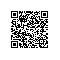# 常见的五类排序算法图解和实现（插入类：直接插入排序，折半插入排序，希尔排序）r2处的38比第一个记录 r1处的49小，那么38插入到49的前面，把插入位置之后的记录顺次的后移一位，同时把带插入记录临时保存在 r0处（复制监视哨）。1 //递增排序
2 void insertSort(int list[])
3 {
4     int j = 0;
5     int i = 0;
6     int temp = 0;
7     //对待排序列直接插入排序
8     //一般把第一个记录先看成是有序子序列，然后从第二个记录开始，逐个的和前面去比较，插入
9     for (i = 1; i <= 8; i++) {
10         //和前面的有序子序列的记录，逐个的比较，直接插入
11         if (list[i] < list[i - 1]) {
12             //复制为监视哨
13             temp = list[i];
14             //移位
15             list[i] = list[i - 1];
16             //继续顺次的和前面的记录进行比较，如果还有不小于它的，那么继续后移，直接插入排序
17             for (j = i - 1; temp <= list[j]; j--) {
18                 //记录后移
19                 list[j + 1] = list[j];
20                 //插入
21                 list[j] = temp;
22             }
23         }
24     }
25 }
26
27 int main(void)
28 {
29     int source = {49, 38, 65, 97, 76, 13, 27, 49};
30
31     insertSort(source);
32
33     for (int i = 0; i < 8; i++) {
34         printf(" %d ", source[i]);
35     }
36
37     return 0;
38 }

13  27  38  49  49  65  76  97 Program ended with exit code: 0mid=（0+6）/2=3，指向39处，20比mid 的值小，插入到 mid 左边，改变 high=mid-1//递增排序
void insertHalfSort(int list[])
{
int j = 0;
int i = 0;
int low = 0;
int high = 0;
int mid = 0;
int temp = 0;//临时监视哨
//一般把第一个记录先看成是有序子序列，然后从第二个记录开始，前面去比较，折半插入
for (i = 1; i <= 8; i++) {
//复制为监视哨
temp = list[i];
low = 0;
high = i - 1;
//当 low>high,则找到了插入位置，为 low或者 high+1
while (low <= high) {
//循环计算 mid 的值
mid = (int)((low + high) / 2);
//待插入记录和 mid进行比较
if (temp < list[mid]) {
//改变 high
high = mid - 1;
}else{
//改变 low
low = mid + 1;
}
}
//循环结束，说明找到了插入位置，进行移位
for (j = i - 1; j >= low; j--) {
list[j + 1] = list[j];
}
//插入
list[low] = temp;
}
}1)、没有除 1 以外的公因子；

2)、最后一个增量值必须为 1。

1)、记录跳跃式前移，在进行最后一趟排序时，已基本有序。

2)、分组后 n 值减小，n^2 更小，而 T(n)=O(n^2)，所以 T(n) 从总体上看是减小了。时间复杂度为 O（n log2（n）），控件复杂度为 o（1）

http://www.cnblogs.com/kubixuesheng/p/4353575.html使用钉钉扫一扫加入圈子
+ 订阅